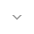belongs to Maven artifact com.android.support:transition:28.0.0-alpha1

# PatternPathMotion

``` public class PatternPathMotion ```
``` ``` ``` extends PathMotion ``` ``` ```

 java.lang.Object ↳ android.support.transition.PathMotion ↳ android.support.transition.PatternPathMotion

A PathMotion that takes a Path pattern and applies it to the separation between two points. The starting point of the Path will be moved to the origin and the end point will be scaled and rotated so that it matches with the target end point.

This may be used in XML as an element inside a transition.

``````<changeBounds>
<patternPathMotion android:patternPathData="M0 0 L0 100 L100 100"/>
</changeBounds>```
```

## Summary

### Public constructors

``` PatternPathMotion() ```

Constructs a PatternPathMotion with a straight-line pattern.

``` PatternPathMotion(Context context, AttributeSet attrs) ```
``` PatternPathMotion(Path patternPath) ```

Creates a PatternPathMotion with the Path defining a pattern of motion between two coordinates.

### Public methods

``` Path``` ``` getPath(float startX, float startY, float endX, float endY) ```

Provide a Path to interpolate between two points `(startX, startY)` and `(endX, endY)`.

``` Path``` ``` getPatternPath() ```

Returns the Path defining a pattern of motion between two coordinates.

``` void``` ``` setPatternPath(Path patternPath) ```

Sets the Path defining a pattern of motion between two coordinates.

### Inherited methodsFrom class ``` android.support.transition.PathMotion ```From class ``` java.lang.Object ```

## Public constructors

### PatternPathMotion

`PatternPathMotion ()`

Constructs a PatternPathMotion with a straight-line pattern.

### PatternPathMotion

```PatternPathMotion (Context context,
AttributeSet attrs)```

Parameters
`context` `Context`

`attrs` `AttributeSet`

### PatternPathMotion

`PatternPathMotion (Path patternPath)`

Creates a PatternPathMotion with the Path defining a pattern of motion between two coordinates. The pattern will be translated, rotated, and scaled to fit between the start and end points. The pattern must not be empty and must have the end point differ from the start point.

Parameters
`patternPath` `Path`: A Path to be used as a pattern for two-dimensional motion.

## Public methods

### getPath

```Path getPath (float startX,
float startY,
float endX,
float endY)```

Provide a Path to interpolate between two points `(startX, startY)` and `(endX, endY)`. This allows controlled curved motion along two dimensions.

Parameters
`startX` `float`: The x coordinate of the starting point.

`startY` `float`: The y coordinate of the starting point.

`endX` `float`: The x coordinate of the ending point.

`endY` `float`: The y coordinate of the ending point.

Returns
`Path` A Path along which the points should be interpolated. The returned Path must start at point `(startX, startY)`, typically using `moveTo(float, float)` and end at `(endX, endY)`.

### getPatternPath

`Path getPatternPath ()`

Returns the Path defining a pattern of motion between two coordinates. The pattern will be translated, rotated, and scaled to fit between the start and end points. The pattern must not be empty and must have the end point differ from the start point.

Returns
`Path` the Path defining a pattern of motion between two coordinates.

### setPatternPath

`void setPatternPath (Path patternPath)`
`patternPath` `Path`: A Path to be used as a pattern for two-dimensional motion.